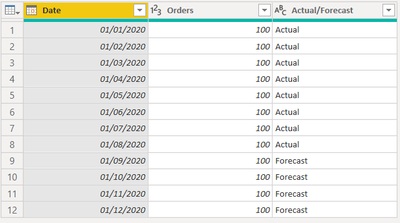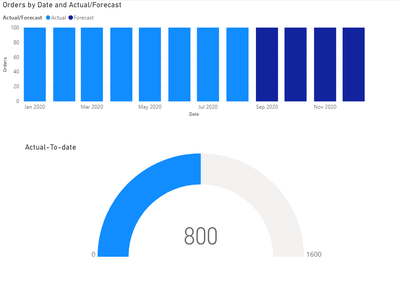cancel
Showing results for
Did you mean:Frequent Visitor

## Visual interactions selected date overruling actual and forecast

I'm having a challenge to find a way to present the Orders to-date on different visuals (bar and Gauge measure).

The solution I'm looking for is to reflect in the Guage visual based on the following:

1. When the report opens (or no filter selected from other visual), Guage presents the Orders based on the Actual table ie. summing all "Actual" orders
2. When I select a certain month on the Stacked Column visual, the Guage gives the accumulated orders till that selected month including Actual + forecastWhat I want, is when I select October the Guage measure will show accumlated sum all the way to October (though it's still forecasted) ie. = 1000. Or selecting December will show the maximum ie. 1200.``````let
Source = Table.FromRows(Json.Document(Binary.Decompress(Binary.FromText("i45WMjDUByIjAyMDJR0lQwMQ6ZhcUpqYoxSrA5E1witrjFfWBK+sKV5ZM7yy5nhlLfDKWqLKuuUXpSYnFpfA5A0NCMgbEpA3wiUfCwA=", BinaryEncoding.Base64), Compression.Deflate)), let _t = ((type nullable text) meta [Serialized.Text = true]) in type table [Date = _t, Orders = _t, #"Actual/Forecast" = _t]),
#"Changed Type" = Table.TransformColumnTypes(Source,{{"Date", type date}, {"Orders", Int64.Type}, {"Actual/Forecast", type text}})
in
#"Changed Type"``````

I've defined 2 DAX measures for the Guage as follows:

``````Actual to-date = CALCULATE(SUM(Monthly_Orders[Orders]), Monthly_Orders[Actual/Forecast] = "Actual", DATESBETWEEN(Monthly_Orders[Date],DATE(2020,1,1), MAX(Monthly_Orders[Date])))

Maximum = CALCULATE(SUM(Monthly_Orders[Orders]), ALL(Monthly_Orders))``````

1 ACCEPTED SOLUTIONSuper User

Hi @Ramiroz ,

Try the following code:

``````Actual-To-date =
IF (
ISFILTERED ( Query1[Date] );
CALCULATE (
SUM ( Query1[Orders] );
FILTER (
ALL ( Query1[Date]; Query1[Actual/Forecast] );
Query1[Date] <= MAX ( Query1[Date] )
)
);
CALCULATE ( SUM ( Query1[Orders] ); Query1[Actual/Forecast] = "ACTUAL" )
)
``````Check PBIX file attach.

Regards

Miguel Félix

Proud to be a Super User!

Check out my blog: Power BI em PortuguêsSuper User

Hi @Ramiroz ,

Try the following code:

``````Actual-To-date =
IF (
ISFILTERED ( Query1[Date] );
CALCULATE (
SUM ( Query1[Orders] );
FILTER (
ALL ( Query1[Date]; Query1[Actual/Forecast] );
Query1[Date] <= MAX ( Query1[Date] )
)
);
CALCULATE ( SUM ( Query1[Orders] ); Query1[Actual/Forecast] = "ACTUAL" )
)
``````Check PBIX file attach.

Regards

Miguel Félix

Proud to be a Super User!

Check out my blog: Power BI em Português